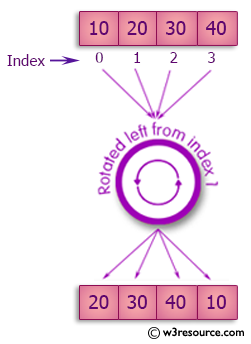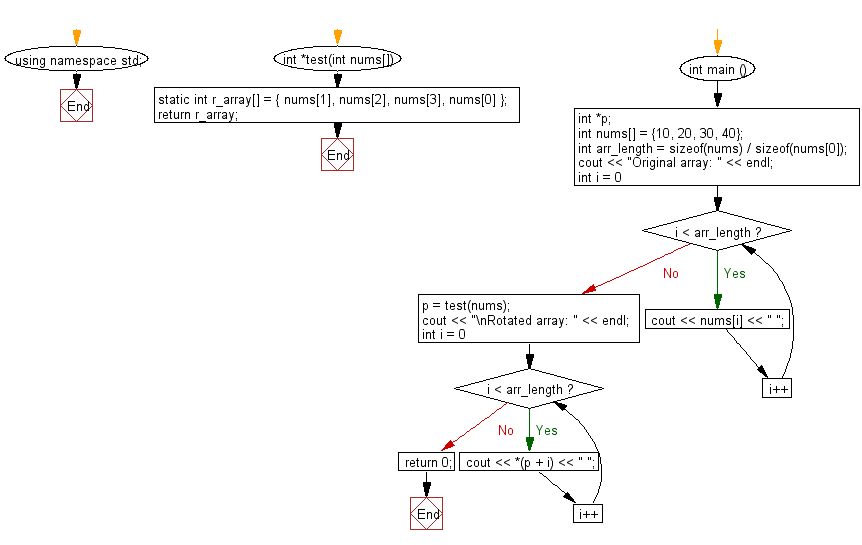﻿ C++ : Rotate the elements of an array in the left direction# C++ Exercises: Rotate the elements of a given array of integers in left direction and return the new array

## C++ Basic Algorithm: Exercise-85 with Solution

Write a C++ program to rotate the elements of a given array of integers (length 4 ) in left direction and return the new array.

Sample Solution:

C++ Code :

``````#include <iostream>
using namespace std;

int *test(int nums[]) {
static int  r_array[] = { nums, nums, nums, nums };
return r_array;
}

int main () {
// a pointer to an int.
int *p;
int nums[] = {10, 20, 30, 40};

int arr_length = sizeof(nums) / sizeof(nums);
cout << "Original array: " << endl;
for ( int i = 0; i < arr_length; i++ ) {
cout << nums[i] << " ";
}

p = test(nums);
cout << "\nRotated array: " << endl;
for ( int i = 0; i < arr_length; i++ ) {
cout << *(p + i) << " ";
}

return 0;
}
``````

Sample Output:

```Original array:
10 20 30 40
Rotated array:
20 30 40 10
```

Pictorial Presentation:Flowchart:C++ Code Editor: